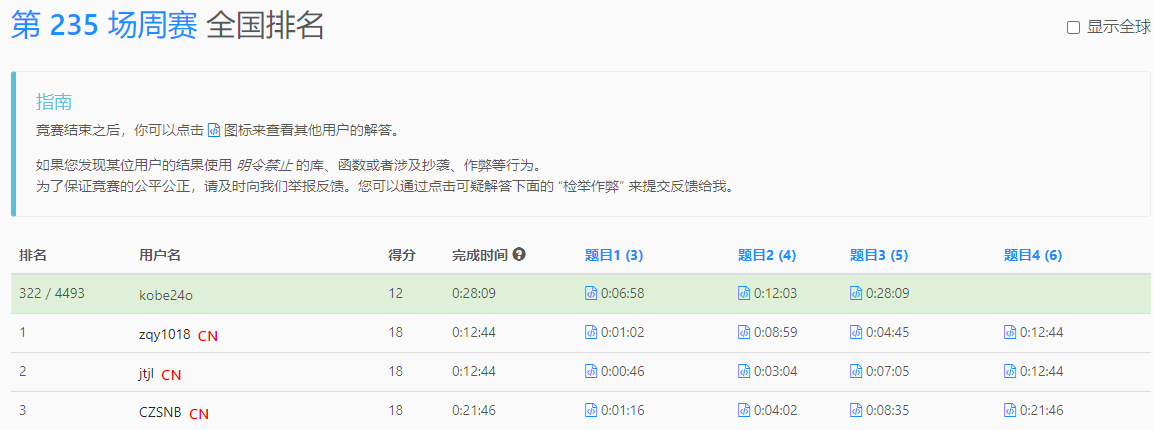# LeetCode 1818. 绝对差值和（二分查找）

### 文章目录## 1. 题目

|x| 定义为：

• 如果 x >= 0 ，值为 x ，或者
• 如果 x <= 0 ，值为 -x
示例 1：

- 将第二个元素替换为第一个元素：[1,7,5] => [1,1,5] ，或者
- 将第二个元素替换为第三个元素：[1,7,5] => [1,5,5]

n == nums1.length
n == nums2.length
1 <= n <= 10^5
1 <= nums1[i], nums2[i] <= 10^5


https://leetcode-cn.com/contest/weekly-contest-235/problems/minimum-absolute-sum-difference/

## 2. 解题

• 对 nums1 排序，遍历 nums2 中的数字 x
• 在 nums1 中二分查找 x 前后的数字，记录最小差值，和 最大的和 的下降量
class Solution {
public:
int minAbsoluteSumDiff(vector<int>& nums1, vector<int>& nums2) {
int n = nums1.size();
long long ans = 0, mod = 1e9+7;
vector<int> t(n);
for(int i = 0; i < n; ++i)
{
ans += abs(nums1[i]-nums2[i]);
t[i] = nums1[i];
}
sort(t.begin(), t.end());
int maxdelta = 0;
for(int i = 0; i < n; ++i)
{
int target = nums2[i], diff = 2e5;
auto it = lower_bound(t.begin(),t.end(),target);
if(it != t.end())
{
diff = min(diff, abs(target-*it)); // 最接近的数的差值
}
auto it1 = upper_bound(t.begin(),t.end(),target);
if(it1 != t.begin())
{
it1--;
diff = min(diff, abs(target-*it1)); // 最接近的数的差值
}
// 对答案缩小有贡献，取最大的
maxdelta = max(maxdelta, abs(nums1[i]-nums2[i])-diff);
}
ans -= maxdelta; //减去最大的缩小值
return ans%mod;
}
};


420 ms 101.7 MB C++08-14483011-201
10-25533
03-22198
06-1254
04-0416
04-055
04-0854
01-1239
09-10614
04-02135
04-0413
02-05250
01-2296
12-1868
01-19132
04-031万+
07-1199
10-30339
©️2020 CSDN 皮肤主题: Age of Ai 设计师:meimeiellie